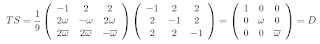occasional meanderings in physics' brave new world

## Thursday, June 19, 2008

### Idempotent Nilpotent II

A nicer way to represent the neutrino tetrahedron group with $3 \times 3$ operators is to choosesince this also obeys $T^{3} = 1$ and $(TS)^{3} = 1$ but $T$ looks a lot more like the circulant $S$ than a diagonal operator. Moreover, the quantum Fourier diagonal still appears in the relationfrom which it also follows that $T = SD$ and $T^{2} = S \overline{D} = S D^{2}$ where $\overline{D}$ is just the rotation in the opposite direction in the plane. Observe how the squaring of $T$ shifts the horizontal phase factors to vertical ones.CarlBrannen said...Kea said...